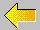# The Universe

Note: This is a copy of part of an unfortunately no-longer on-line webage from the National Solar Observatory. For more information about the size of the known universe check the Wikipedia entry on the: Observable Universe.

## Mass, Size, and Density of the Universe

The mass, size, and density of the universe involve very big and very small numbers with large numbers of zeros in front of or behind the decimal point. Scientists write such numbers using a special code that keeps them short. When they write a number like 6e7 then the number after the "e" indicates by how many places the decimal point must be shifted to the right. For instance, 6e7 is equal to a 6 with 7 zeros behind it, or 60,000,000, and 6.1e7 is equal to 61,000,000. If the number after the "e" is negative, then the decimal point is shifted to the left. For instance, 6e-3 is equal to a 6 with 3 zeros in front of it (and the decimal point after the first zero), or 0.006, and 6.1e-3 is equal to 0.0061.

The mass density of visible matter (i.e., galaxies) in the Universe is estimated at 3e-28 kg/m^3 (3e-31 times the mass density of water). The radius of the visible Universe is estimated at 1.7e26 m (18 thousand million lightyears) plus or minus 20 percent or so. This yields a total mass of the visible matter of about 6e51 kg (1.3e52 lb), which is equivalent to the weight of 4e78 hydrogen atoms. Since nine out of ten atoms and ions in the Universe are in the form of hydrogen, this is a reasonable estimate for the number of atoms in the Universe (based on the visible galaxies only). Maybe a correction factor of the order of 2 has to be applied to account for the warping of space on very large scales.

However, there is considerable uncertainty about the mass density of all matter (visible and invisible) and energy (through Einstein's E = mc^2 equation). When one studies the movement of matter in and around galaxies, then it appears that up to about 10 times more mass is pulling at the matter (through its gravity) than is accounted for in the visible stars. This is the "missing-mass" problem. If this factor of ten holds throughout the Universe, then the total mass in the Universe would be about 6e52 kg. If the missing mass were mostly in the form of hydrogen atoms (which is not at all clear) then the number of atoms would be about 4e79.

A currently popular theory of the formation of the Universe (the so-called Inflation Theory) predicts that the mass density of the Universe should be close to the so-called critical density that separates an open universe that always grows from a closed universe that ultimately collapses again. This critical mass density is currently equal to 6e-27 kg/m^3. If the Universe is at the critical density, then the total mass of the Universe is closer to 1e53 kg, and the number of atoms (assuming that most of the mass is in the form of hydrogen atoms) about 6e79.

It seems, then, that the number of atoms in the Universe is at least about 4e78, but perhaps as many as 6e79. I would suggest 1e79 as a reasonable estimate. That is, 10 000 000 000 000 000 000 000 000 000 000 000 000 000 000 000 000 000 000 000 000 000 000 000 000 000 000 atoms.

Of course, besides material particles there are also lots of photons and neutrinos flying around the Universe. It is estimated that there are about 1e9 times as many photons and neutrinos as atoms in the Universe.Return To Sunspot Astronomy and Visitor Center Home Page